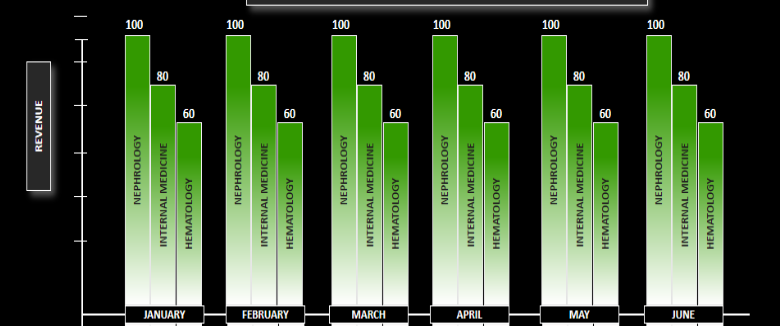QlikView Creating Analytics

Discussion Board for collaboration related to Creating Analytics for QlikView.

Announcements
QlikWorld 2020: Join us May 11 - 14, 2020 in Phoenix, AZ. Register early and save \$400. Learn More
New Contributor

Top three values per month

I'm trying to build a bar chart (using a standard Qlik sense component or the Picassa extension).

I need 3 bars PER MONTH that represent my top 3 categories per month based on total revenue of that category.While my sample chart does not show it, the top 3 categories will be different every month.

Best I can tell, I need to make 3 data series

=Dual(Aggr( If(Rank(Sum(REVENUE))=1, CATEGORY_NAME),  MonthYear, PHY_SPEC_DESCR),

Sum( Aggr( If(Rank(Sum(REVENUE))=1, Sum(REVENUE)),  MonthYear, CATEGORY_NAME))

)

=Dual(Aggr( If(Rank(Sum(REVENUE))=2 CATEGORY_NAME),  MonthYear, PHY_SPEC_DESCR),

Sum( Aggr( If(Rank(Sum(REVENUE))=2, Sum(REVENUE)),  MonthYear, CATEGORY_NAME))

)

=Dual(Aggr( If(Rank(Sum(REVENUE))=3, CATEGORY_NAME),  MonthYear, PHY_SPEC_DESCR),

Sum( Aggr( If(Rank(Sum(REVENUE))=3, Sum(REVENUE)),  MonthYear, CATEGORY_NAME))

)

Is there a simpler way to do this?  I'm not confident that I'm getting the right data out.

Thanks, Mark

1 Solution

Accepted Solutions
Highlighted
Honored Contributor